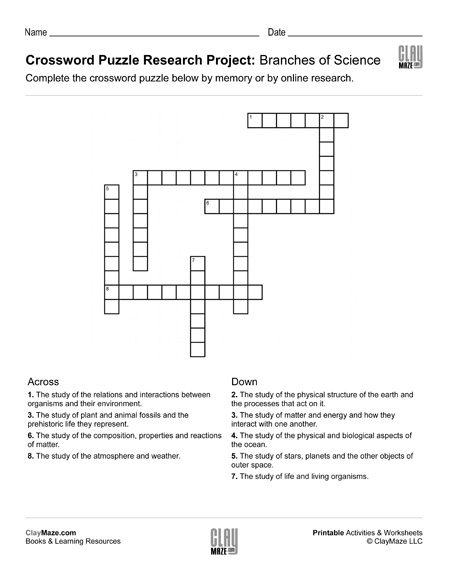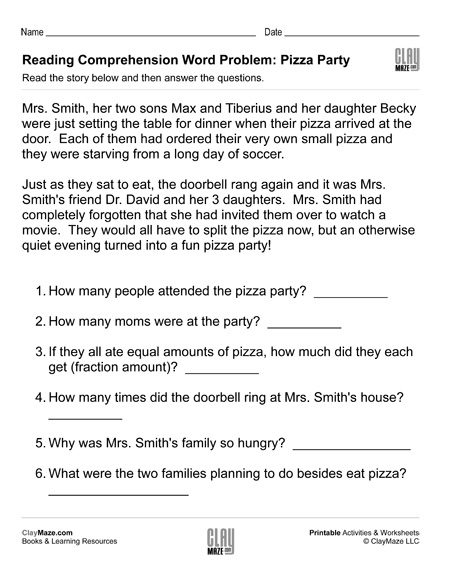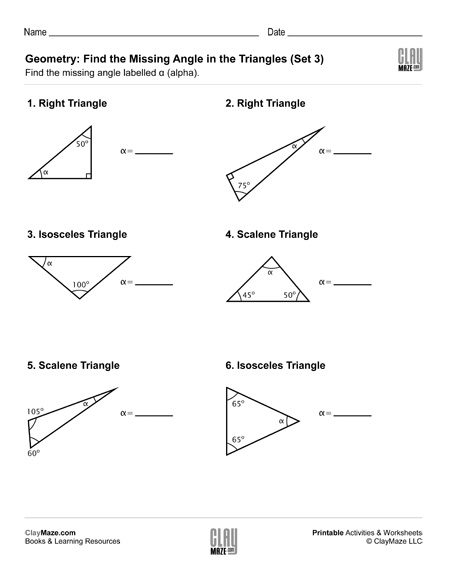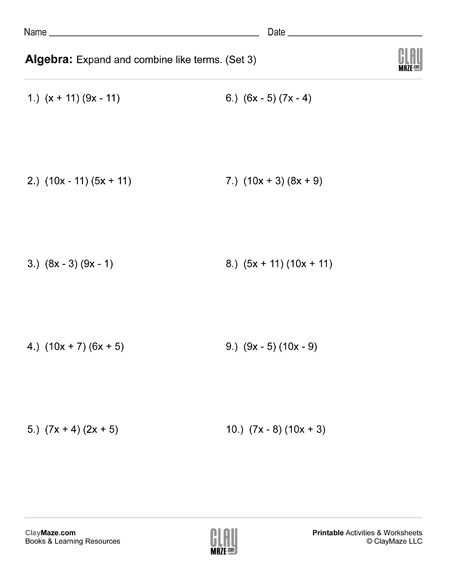Free Printable Worksheets for Fifth Grade

Crossword Puzzle Research Project – Branches of ScienceThis is a little bit of a spin from a traditional Crossword Puzzle lesson.   This worksheet can be used as a followup to some study on the branches of science or it can be used as an online researc...

Reading Comprehension Word Problem: Pizza PartyAs our subject matter becomes more and more technical and complex, it becomes increasingly important for children to learn to read carefully and re-read a passage in order to fully grasp the...

Solve the Equations I (Set 1)This is a worksheet for students learning how to solve equations. Each problem has a combination of multiplication and either addition or subtraction, but division will also need to be used to...

Solve the Equations I (Set 2)Download this worksheet for practice in solving equations. The student is asked to find the value of x in each of the problems....

Solve the Equations I (Set 3)Print this practice worksheet for students learning to solve equations. Each problem has a combination of multiplication and either addition or subtraction. Division will also be used to find the...

Solve the Equations I (Set 4)Use this worksheet for practice with solving equations. Solve for x in each problem. – 15 problems...

Geometry: Find the Missing Angle in the Triangle (Set 1)This worksheet is for finding a missing angle in a triangle and is based on the student knowing that there are 180 degrees in a triangle. It also assumes the student knows the different types of...

Geometry: Find the Missing Angle in the Triangle (Set 2)Download this worksheet on finding the missing angle in a triangle. This worksheet assume the student is familiar with right, isosceles and scalene triangles and knows that the angles in a...

Geometry: Find the Missing Angle in the Triangle (Set 3)Geometry worksheet on triangles and angles. The student is asked to find the unknown angle using their knowledge of triangles....

Geometry: Find the Missing Angle in the Triangle (Set 4)In this worksheet the student is asked to find the missing angle in the triangles shown. This sheet is for students that are familiar with right, scalene and isosceles triangles....

Reading Comprehension Word Problem: Lab Experiment 3This free children’s worksheet is the 3rd part of a 3 part series which combines scientific experiment with word problems.  Exercises like these are a great bridge between early learning...

Algebra: Expand & Combine Like Terms (Set 1)Use the worksheet for practice multiplying expressions and combining like terms....

Algebra: Expand & Combine Like Terms (Set 2)Algebra worksheet on multiplying expressions in parentheses and combining like terms....

Algebra: Expand & Combine Like Terms (Set 3)